# Excel in formula builder

How to upload your excel and use it in the formula builder

When you are building a calculator and you have already done calculations in an excel, you can either translate this excel formula onto our platform directly in the formula builder as seen here or simply upload an excel and follow the steps mentioned below:

# Use Excel based Table

Once you are in the formula builder, you need to click on the second tab on top right- Use Excel Based Table to upload your excel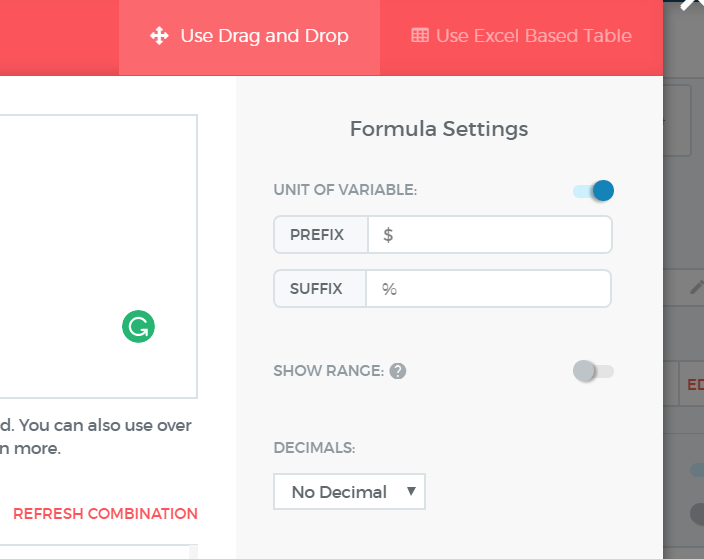Click on upload excel to find your excel in the formula builder.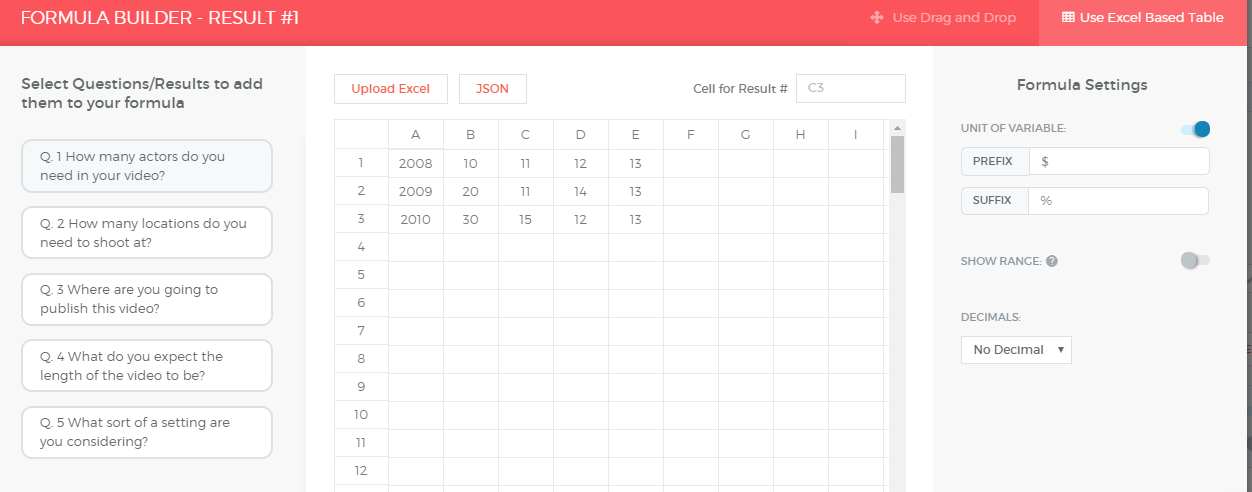After you have uploaded the excel, you need to map your excel question cells with the question numbers of the calculator you are creating.

For example, here cell B2 is for question number 1, then you need to insert =Q_1 in the cell. Repeat this for all the questions that are used in the final result formula.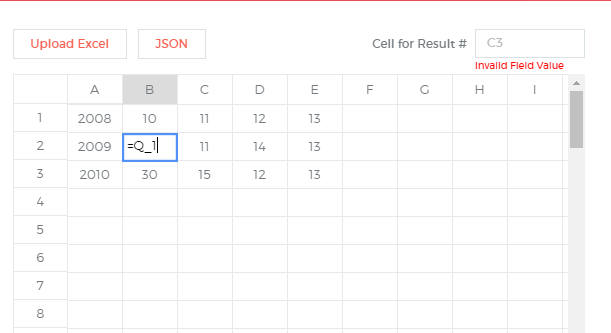### 📘Note:

Any cell that needs to reference a question should be of the format =Q_1 for question 1, and any cell that references a cell (for fixed values) can just be =A1+B1.

# Map the Result

After you have mapped all the questions in the excel, you need to finally map the cell number for the result in the space provided at the top. In this case, cell B4 is used for calculating the Result 1.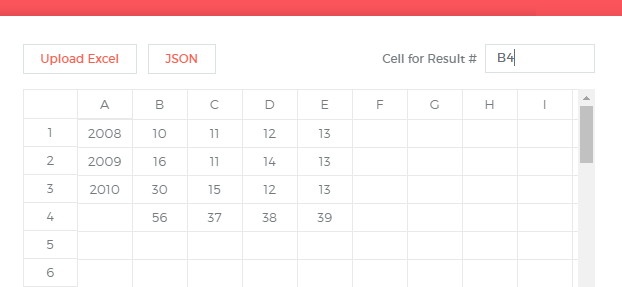Once you are done mapping all the required cells with the questions and results of the quiz, click on apply excel formula on bottom right and exit. You need to repeat this for all the other results separately if you have multiple results to display.

# Using if_else in the excel

You can use if else conditions similar to the one you use directly in the excel in the format of IF(condition, true, false). In the example below, we have used the condition that if B3 is greater than B2, then Q1*10 else Q1.

To use this condition in the excel, you insert in the cell: IF(B3>B2,Q_1*10,Q_1)

Whereas to use the same condition directly in the formula builder where B2 and B3 represent question 2 and 3 respectively, you write: Q3>Q2?Q1*10:Q1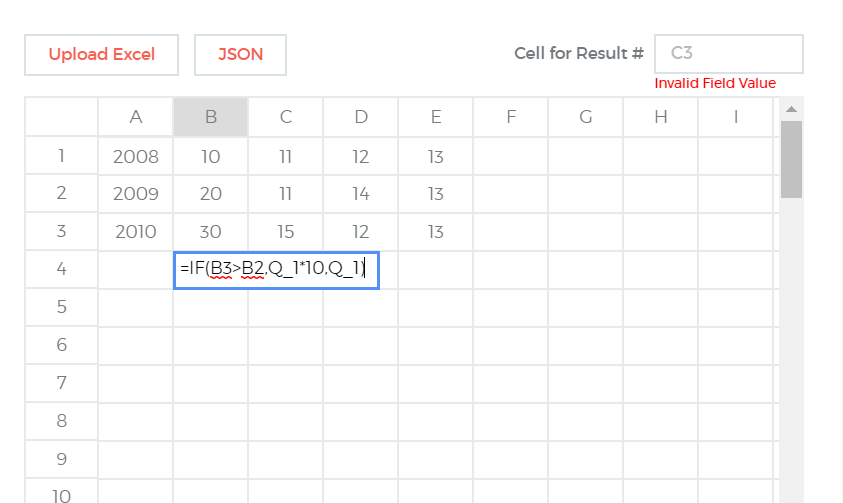### 📘Nested IF:

If you have multiple IF-ELSE conditions, you can use the following format -
IF(condition,true,(IF(condition,true,IF(condition,true,false))))

For example, If question 1 is equal to 0, then it should be 1 else if question 1 is greater than equal to 2, then Q1*10 else 1.

IF(Q_1==0,0,(IF(Q1>=2,Q_1*10,1)))

For inserting nested IF conditions in the builder directly, you can refer more details on this link.

Hope this helps. If you still have questions, feel free to use our chat widget on the bottom right to get in touch with our excel and maths experts :)